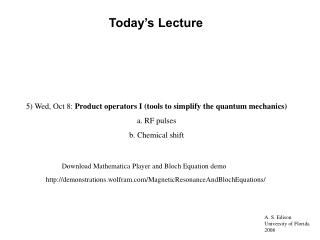# Today’s Lecture - PowerPoint PPT PresentationDownload PresentationToday’s Lecture

Today’s Lecture
Download Presentation## Today’s Lecture

- - - - - - - - - - - - - - - - - - - - - - - - - - - E N D - - - - - - - - - - - - - - - - - - - - - - - - - - -
##### Presentation Transcript

1. Today’s Lecture 5) Wed, Oct 8: Product operators I (tools to simplify the quantum mechanics) a. RF pulses b. Chemical shift Download Mathematica Player and Bloch Equation demo http://demonstrations.wolfram.com/MagneticResonanceAndBlochEquations/

2. We’ve really just been talking about rotations most of the time! NMR is all rotations.

3. Vector representations of Mx, My, and Mz x y Notice that the coordinate system satisfies the “right hand rule”. If you point your right thumb along the z-axis, you fingers will close from x to y. z x y z x y z

4. Rotation Matrices These are 3D rotation matrices. When they act on a vector, they rotate the vector around the axis that defines the vector (Rx around x; Ry around y; Rz around z).

5. What is a rotation? Here is an example of a rotation of Mz around the x-axis by an angle f: Notice that if f=0, the vector stays the same. If f=p/2, the vector is rotated to the –y axis.

6. General Rule of Rotations Every rotation in 3D space leads to 2 terms. The first term is pointing in the same direction and is multiplied by Cos(f) where f is the rotation angle. The second term is along the axis that the vector is rotated into and is multiplied by Sin(f). For example, in the rotation above, a rotation of f around the x-axis (because it is the Rx rotation matrix) of Mz produces Mz*Cos(f)-My*Sin(f).

7. Product Operators are a mathematical shorthand to do rotations. For example, the rotation we just saw: becomes:

8. Product Operator Rules • The thing above the arrow is the operator. The one shown above is a f pulse along the positive x-axis. The nucleus that we are worrying about is given by a capital letter like “I”. The orientation of the magnetization is given by the subscript. If we just have one nuclear spin to worry about (e.g. water), then there are 3 spatial orientations for that nucleus: • Iz (pointing along the z-axis) • Ix (pointing along x) • Iy (pointing along y)

9. Product Operator Rules Just like a pulse that produces a torque, operators rotate around a given axis but do not act on things along the axis of rotation. Corresponding to B1 fields along the x and y axes, we have 2 operators for pulses: Pulse along the x-axis by f degrees Operators have hats. Pulse along the y-axis by f degrees

10. Examples of pulses

11. What is a rotation around the z-axis? Chemical shift! The chemical shift operator works exactly like a pulse operator, but it only acts along the z-axis. The only difference between the convention for a pulse and chemical shift is that we put in the frequency (W) times time for the shift and only the rotation angle for the pulse.

12. 1D NMR experiment a a b c (+ relaxation) a b c

13. Product Operator I Summary • Operators act on magnetization vectors and cause rotations. • Rotations always have the starting term multiplied by cosine and the resulting term multiplied by sine of the angle. • We have discussed two kinds of operators, pulse and chemical shift. These both do “normal” rotations in 3D and leave the magnetization unaltered. • Operators can act sequentially (e.g. pulse followed by evolution). This becomes critical in analyzing 2D or multiple pulse experiments.

14. Next Lecture 6) Fri, Oct 10: Product operators II a. Scalar (J) coupling b. Multiple pulse experiments